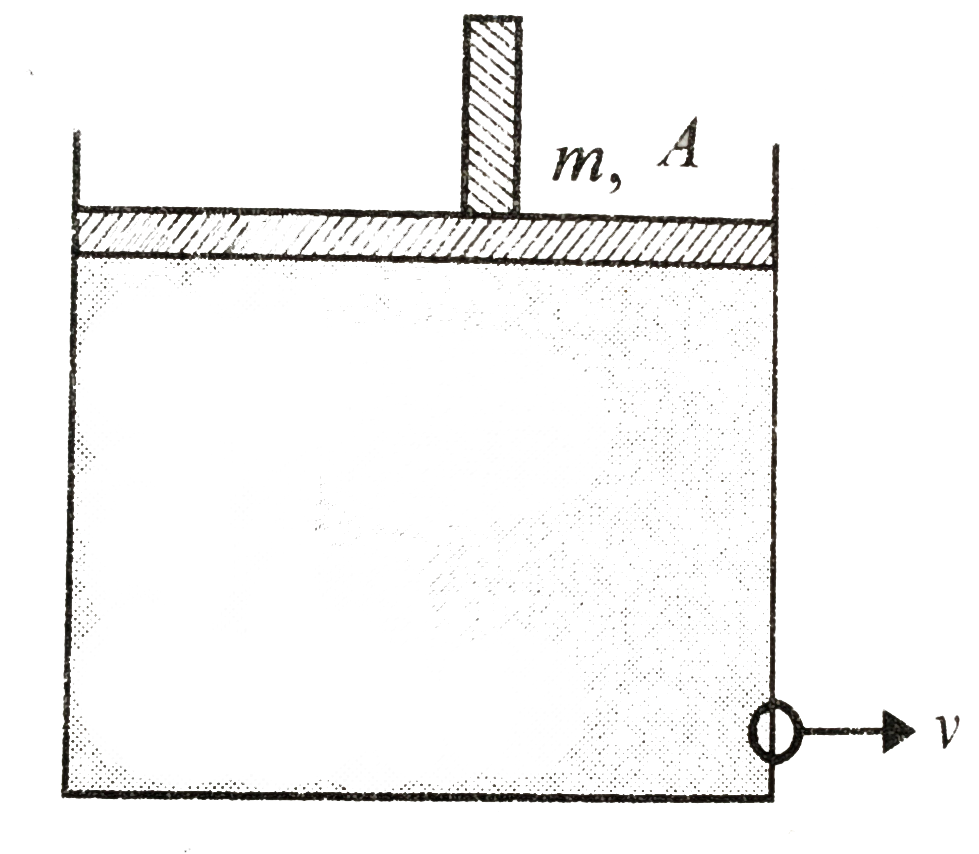Doubtnut App - Get 50 Lakh+ video solutions better than GoogleInstall Now

# A cylindrical vessel contains a liquid of density ρ up to height h. The liquid is closed by a piston of mass m and area of cross section A. There is a small hole at the bottom of the vessel. The speed v with which the liquid comes out of the hole isA

2gh

B

2(gh+mgρA)

C

2(gh+mgA)

D

2(gh+mgA)

Text Solution
Verified by Experts

## Applying Bernoulli's theorem at point 1 and 2 difference in pressure energy between 1 and 2= difference in kinetic energy between 1 and 2.Here ρhg=mgA=12ρv2 or v= ⎷(2gh2mgρA)=√2(gh+mgρA)